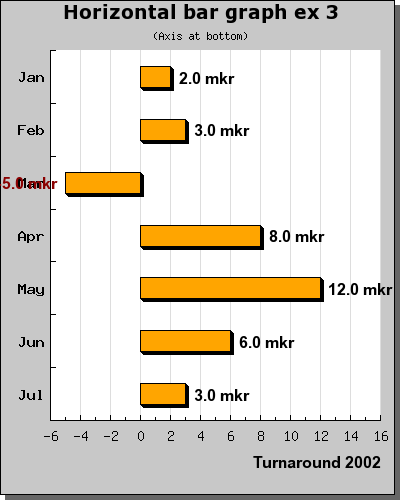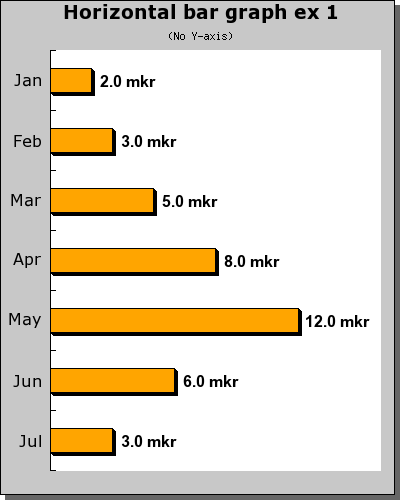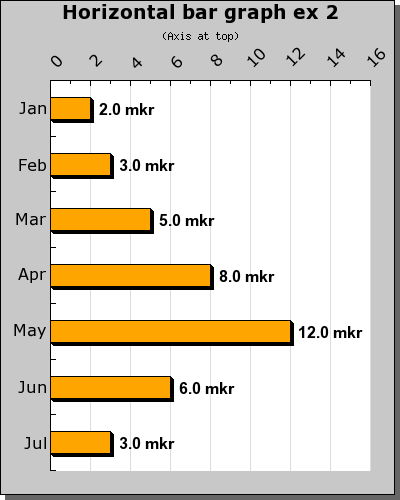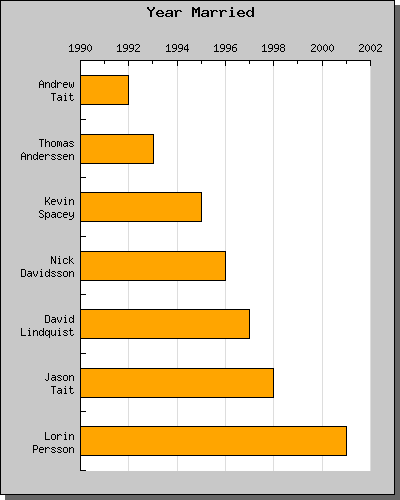Contents Previous Next

### 8.2.8 Horizontal bar graphs

It can often come in handy to have horizontal bar graphs especially if you have a large number of values to display. Even though JpGraph doesn't directly support horizontal bar graphs this is easy achieved by constructing a normal vertical bar graph which is then rotated 90 degrees.

The example below shows a simple example of thisFigure 40: A typical horizontal bar graph with the Y-axis at the bottom [src]

In order to achieve this effect you should study the above example carefully and you might notice two things

• We don't simply rotate the graph we also specify that we want the rotation center to be the middle of the entire image. The reason for this is that by default (See the section on rotating plots) the pivot point for rotation is the center of the plot area. Since the center of the plot area is not necessary the center of the entire image the rotation might be a little bit difficult to predict since it will depend on the margins specified. <
• The size of the plot area is determined from the original width and height of the image taking the specified margin into account. When the the plot area is rotated 90 degrees clockwise what was the left margin now in effect become the upper margin and so on. This is a small nuance since we conceptually want to specify the margins directly in the rotated plot.
In order to more easily handle the margin with rotated images it is recommended that the method "Set90AndMargin()" is used. This allows the image to be rotated as well as specifying the margins in an obvious way.

We finally show three more examples of horizontal bar plots. In the first plot we have hidden the Y-axis and in the second we have positioned the Y - axis at top as opposed to the bottom as the first example shows.Figure 41: Horizontal bar graph with hidden Y axis [src]Figure 42: Horizontal bar graph with Y axis at the top [src]

In the final example which is almost similar to the two first we illustrate the use of labels with more than one line.Figure 43: Horizontal bar graph with manual integer scale as well as multiple line labels [src]

Contents Previous Next